Transformations: Reflections MathBitsNotebook.com Terms of Use   Contact Person: Donna RobertsYou are familiar with the term reflection. You see reflections every day.

A reflection can be seen, for example, in water, a mirror, or in a shiny surface.
Take a look at the following reflections.
 Reflection in Water Reflection in a Mirror Reflection in Shiny Surface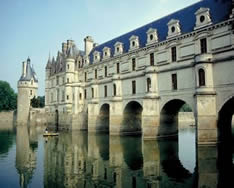A reflection of an object is the object as it would be seen in a mirror.
In a mirror image, your left and right are reversed. Your image has been "flipped".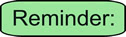Reflections are FLIPS!!

Reflection in a Line:
 A reflection can be thought of as folding or "flipping" an object over the line of reflection. • The original object is called the pre-image, and the reflection is called the image. • The image is usually labeled using a prime symbol, such as A'B'C'. • An object and its reflection have the same shape and size, but the figures face in opposite directions. The objects appear as if they are mirror images, with right and left reversed.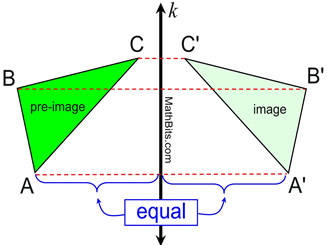• The object and its reflection are the same distance from the line of reflection, line k. The line of reflection is the perpendicular bisector of the segment joining every point and its image point. • Since under a reflection, the figures remain congruent (same size and shape), a reflection is called a rigid transformation and is said to preserve length. If AB = 6 inches, then under a reflection, A'B' = 6 inches. • Also, naming (lettering) the figure in a reflection requires changing the order of the letters (such as from clockwise to counterclockwise).
 Properties preserved under a line reflection from the pre-image to the image. 1. distance (lengths of segments remain the same) 2. angle measures (remain the same) 3. parallelism (parallel lines remain parallel) 4. collinearity (points remain on the same lines) ---------------------------------------------------------- The orientation (lettering around the outside of the figure), is not preserved. The order of the lettering in a reflection is reversed (from clockwise to counterclockwise or vice versa).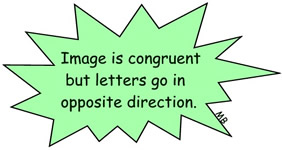The preserved properties of a transformation are referred to as being "invariant" in that they do not vary (change) during the transformation.Reflections in the coordinate plane:Reflect over the x-axis: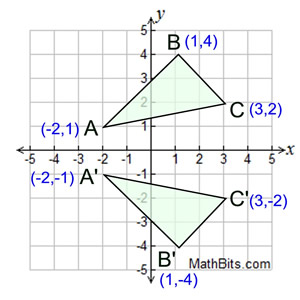When you reflect a point across the x-axis, the x-coordinate remains the same, but the y-coordinate is transformed into its opposite (its sign is changed).

If you forget the rules for reflections when graphing, simply fold your paper along the x-axis (the line of reflection) to see where the new figure will be located.

Or you can measure how far your points are away from the x-axis to locate the new points, such as B is 4 vertical units above the x-axis, so B' will be 4 vertical units below the x-axis.

 The reflection of the point (x,y) across the x-axis is the point (x,-y). (opposite sign for y-coordinate)
notation:    rx-axis (x,y) = (x,-y)Reflect over the y-axis: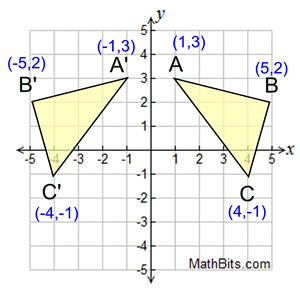When you reflect a point across the y-axis, the y-coordinate remains the same, but the x-coordinate is transformed into its opposite (its sign is changed).

Notice that B is 5 horizontal units to the right of the y-axis, and B' is 5 horizontal units to the left of the y-axis.

 The reflection of the point (x,y) across the y-axis is the point (-x,y). (opposite sign for x-coordinate)
notation:    ry-axis (x,y) = (-x,y)Reflect over y = x: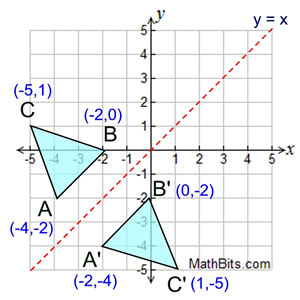When you reflect a point across the line y = x, the x-coordinate and y-coordinate change places. If you reflect over the line
y = -x, the x-coordinate and y-coordinate change places and are negated (the signs are changed).
 The reflection of the point (x,y) across the line y = x is the point (y, x). (swap x and y coordinates)
notation:    ry=x (x,y) = (y,x)

 The reflection of the point (x,y) across the line y = -x is the point (-y, -x). (swap x and y coordinates and give opposite signs)
notation:    ry=-x (x,y) = (-y,-x)Reflect over any line: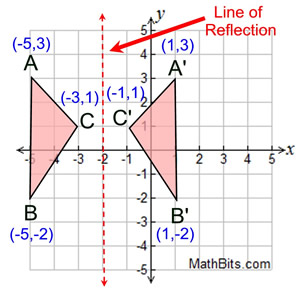Remember that each point of a reflected image is the same distance from the line of reflection as the corresponding point of the original figure. The line of reflection will lie directly in the middle between the original figure and its image.

 Notice that each point of the original figure and its image are the same distance away from the line of reflection. You may be able to simply "count" these distances on the grid.A small plastic device, called a Mira™ , can be used when working with line reflections. The Mira is placed on the line of reflection and the original object is reflected in the plastic. By looking through the plastic, you can see what the reflection will look like on the other side and you can trace it with your pencil.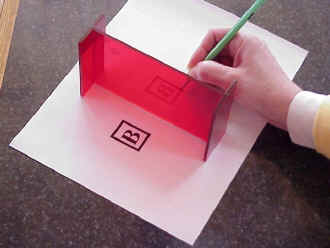Reflection in a Point:

 A point reflection exists when a figure is built around a single point called the center of the figure, or point of reflection.  For every point in the figure, there is another point found directly opposite it on the other side of the center such that the point of reflection becomes the midpoint of the segment joining the point with its image.  Under a point reflection, figures do not change size or shape.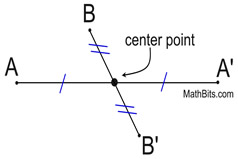While any point in the coordinate plane may be used as a point of reflection, the most commonly used point is the origin. Assume that the origin is the point of reflection unless told otherwise.Reflect in origin (0,0):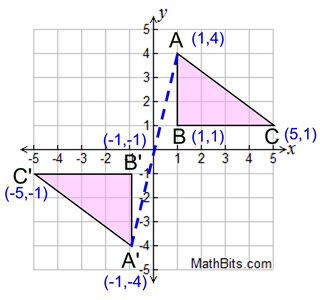Triangle A'B'C' is the image of triangle ABC after a point reflection in the origin.

Imagine a straight line connecting A to A' where the origin is the midpoint of the segment.

When you reflect a point in the origin, both the x-coordinate and the y-coordinate are negated (their signs are changed).

 In a point reflection in the origin, the image of the point (x,y) is the point (-x,-y).

 Properties preserved under a point reflection from the pre-image to the image. 1. distance (lengths of segments remain the same) 2. angle measures (remain the same) 3. parallelism (parallel lines remain parallel) 4. collinearity (points remain on the same lines) 5. midpoint (midpoints remain the same in each figure) 6. orientation (lettering order remains the same)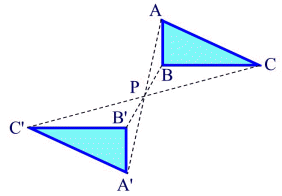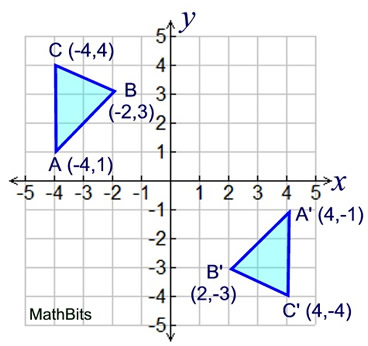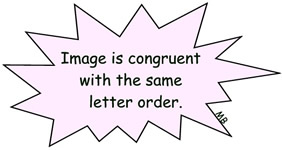Notice how the coordinates of triangle A'B'C' are the same coordinates as triangle ABC,
BUT the signs have been changed.

Triangle ABC has been reflected in the origin.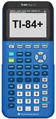For calculator help with transformations click here.